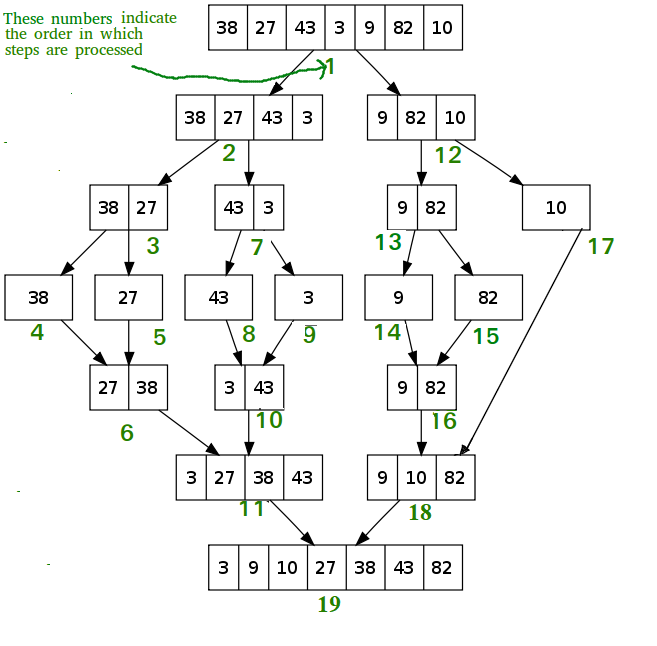# Merge Sort Algorithm | C Programming | Factsprime – DSA

### Write a Modular C Programming code for ‎Merge Sort Algorithm DSAMerge sort is a sorting algorithm that works by dividing an array into smaller subarrays, sorting each subarray, and then merging the sorted subarrays back together to form the final sorted array.

CODE:

```#include<stdio.h>

void printArray(int A[], int n)
{
for (int i = 0; i < n; i++)
printf("%d ", A[i]);

printf("\n");
}

void merge(int A[], int mid, int low, int high)
{
int i, j, k, B;
i = low;
j = mid + 1;
k = low;

while (i <= mid && j <= high)
{
if (A[i] < A[j])
B[k++] = A[i++];

else
B[k++] = A[j++];

}
while (i <= mid)
B[k++] = A[i++];

while (j <= high)
B[k++] = A[j++];

for (int i = low; i <= high; i++)
A[i] = B[i];
}

void mergeSort(int A[], int low, int high){
int mid;
if(low<high){
mid = (low + high) /2;
mergeSort(A, low, mid);
mergeSort(A, mid+1, high);
merge(A, mid, low, high);
}
}

int main()
{
int a,n;

printf("Enter the size: ");
scanf("%d",&n);

printf("Enter Numbers: ");
for(int i=0;i<n;i++)
a[i]=(rand()%10);

printArray(a, n);
mergeSort(a, 0, n-1);
printf("Sorted array is: ");
printArray(a, n);

}

```

OUTPUT

```Enter the size: 26
Enter Numbers: 1 7 4 0 9 4 8 8 2 4 5 5 1 7 1 1 5 2 7 6 1 4 2 3 2 2
Sorted array is: 0 1 1 1 1 1 2 2 2 2 2 3 4 4 4 4 5 5 5 6 7 7 7 8 8 9

Process returned 0 (0x0)   execution time : 1.121 s
Press any key to continue.```

Please find some more codes of Loops, Condition Statements, 1D Arrays, 2D Arrays, Strings, Pointers, Data Structures, Files, Linked lists, Stacks, Queues, Binary Trees, MISC, Solved model question papers & Hacker Rank all solutions on the below page:

Top 100+ C Programming codes – KLE Technological University